# Box 1

In order to deal quantitatively with clocks synchronization it is customary to use a formalism developed by Hans Reichenbach in 1928. In the following picture the typical synchronization scene is depicted.When clock A reads time tA=t1 a light signal is sent towards clock B, which is reached at time tB=t2. The light signal is instantly reflected towards A which, in turn, is reached at tA=t3. Using Einstein’s convention we are entitled to define t2 as: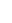This definition seems quite natural. After all, the velocity of light is constant and the time it takes to go from A to B equals the time taken for the reverse journey. But we have seen that a one-way velocity is in principle non-measurable unless a synchronization procedure is first selected. Thus, another valid choice for time t2 is the following: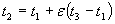,                  (1.1)

ε being a real number between 0 and 1 (0<ε<1). Each numerical value assumed by ε corresponds to a specific synchronization or, equivalently, to a specific value for the one-way velocity of light. When ε=1/2 formula (1.1) becomes Einstein’s synchronization.

Let us, now, find an expression for the one-way velocity of light from A to B, indicated as cε+, as function of the two-way velocity of light, c, and the synchronization parameter ε. The two-way speed of light is a quantity that can be measured without any ambiguity because it is carried out with only one clock; therefore there is no need to synchronize distant clocks. Put simply, when the light signal is sent from A to B and instantly reflected to A, we define c as: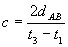(1.2)

To measure one-way velocities we use two synchronized clocks positioned at A and B. Then cε+ is defined as follows:From (1.1) we know that t2-t1=ε(t3-t1), then the above expression is transformed into the following one: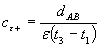Using formula (1.2), t3-t1 can be expressed as 2dAB/c. Thus, finally,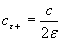(1.3)

Formula (1.3) clearly shows how assigning a given synchronization (i.e. a particular value of ε) is equivalent to defining a particular one-way velocity of light. This makes sense because, according to the arguments previously introduced, one-way velocities contain a non-eliminable element of conventionality. Incidentally, if we use Einstein’s synchronization, ε=1/2, in formula (1.3), obtain cε+=c; this is in agreement with Einstein’s postulate that the one-way velocity of light equals c.

The velocity of light is not the only velocity affected by a synchronization choice. Indeed all one-way velocities will strongly depend upon the particular way distant clocks are synchronized. An expression for any one-way velocity, vε+, can be obtained with the procedure described hereafter. Consider, again, clock A and clock B in the following picture.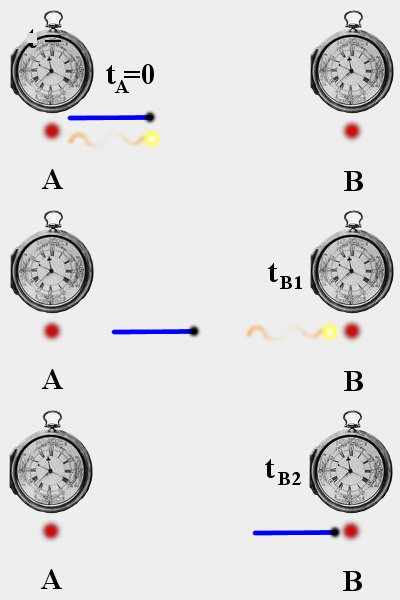At tA=0 both a light signal and a point-like body leave A and travel towards B. Light will, eventually, reach B at tB1=dAB/c, if Einstein’s synchronization is used, at tB1=dAB/cε+, if any other synchronization is used. Similarly, the body will reach B at tB2=dAB/v, using Einstein’s synchronization, or at tB2=dAB/vε+, using any other synchronization. But, no matter what synchronization has been selected, the difference tB2-tB1 will always be the same, because the unit of time is assumed to be the same for all clocks. In formula: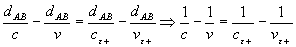cε+ can be replaced using formula (1.3). After some algebraic manipulation the above equation yields the following expression for vε+: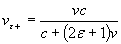(1.4)

Equation (1.4) leaves no doubt on the dependency of any one-way velocity from the synchronization parameter. When Einstein’s synchronization is adopted, ε=1/2, vε+ reduces to v which, quite appropriately, is called the two-way velocity of the point-like body.